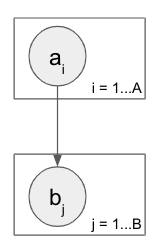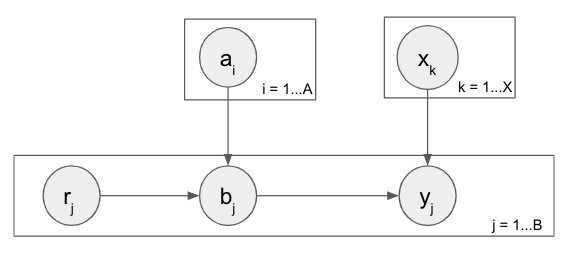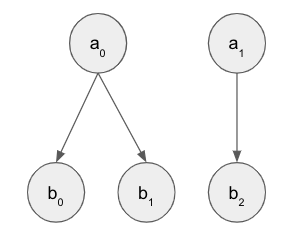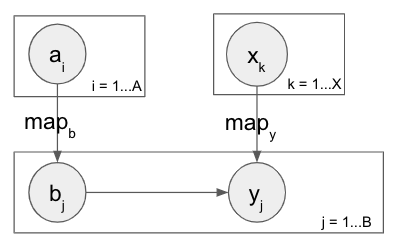# Using parallel plates during discrete enumeration

Now that I have MAP prediction working thanks to help from this post I’m expanding my toy model closer to my actual application, but I have some followup questions

`a` is still Bernoulli and `b` is still a mixture of Bernoullis given `a`, and are very likely to take the value of `a`. But now instead of belonging to nested plates they’re in separate plates, and there is a new mapping, eg `M = [0 0 1 0 1]` that denotes which `a_i` dictates the mixture for each `b_j`. This is kind of unconventional I think but needed for further expansion towards my application (explained in the digression at the bottom).I’ve been able to get this working easily enough with sequential plates. The toy dataset has `a = [0, 1]` and the first five `b` belong to the first `a` and are thus 0, and vice versa for the second five `b`. With this setup I’m able to correctly predict `a` given `b` and also `b` given `a`.

MWE w/ sequential plates
``````import torch
import pyro
import pyro.distributions as dist
from pyro.infer import infer_discrete, config_enumerate
from pyro.ops.indexing import Vindex
import pyro.optim
from pyro import poutine

pyro.enable_validation(True)

num_b = 10

a_cpd = torch.tensor(0.9)
b_cpd = torch.tensor([.01, .99])

# first half of a and b are 0, second half are 1
data = {}
data['a'] = torch.tensor([0., 1.])
data['b'] = torch.cat((torch.zeros(num_b//2), torch.ones(num_b//2)))
data['map'] = torch.cat((torch.zeros(num_b//2), torch.ones(num_b//2)))

@config_enumerate
def model(a_obs=None, b_obs=None):

# sample a
a = []
for i in pyro.plate("plate_a", size=2):
a.append(pyro.sample(f'a_{i}',
dist.Bernoulli(a_cpd),
obs=a_obs[i] if a_obs is not None else None
))

# sample b
for i in pyro.plate("plate_b", size=num_b):
a_ind = int(data['map'][i])
a_cat = a[a_ind].long()
pyro.sample(f'b_{i}',
dist.Bernoulli(Vindex(b_cpd)[a_cat]),
obs=b_obs[i] if b_obs is not None else None
)

inferred_model = infer_discrete(model, temperature=0, first_available_dim=-2)

for target_var in ["a", "b"]:
kwargs = {"a_obs": data["a"].float()} if target_var == "b" else {"b_obs": data["b"].float()}
trace = poutine.trace(inferred_model).get_trace(**kwargs)
for key, val in sorted(trace.nodes.items()):
if key == target_var:
print(key, val["value"])
``````

The issue is that `B` could get very large, and the model itself will grow too, so the exponential runtime explosion for discrete enumeration with sequential plates becomes prohibitive. Given Restriction 2 for parallel plates used in discrete enumeration, I don’t expect to be able to parallelize the `a_plate`. But as far as I can tell I should be able to do so for the `b_plate`.

With a parallel `b_plate` I can get the prediction working for `b` given `a`, but can’t do it for predicting `a` given `b`. The issue comes in the call to `model` when `a` is enumerated, in which case I can’t figure out how to properly construct the `mapped_cpd` that compiles the appropriate cpd for each `b` based on its corresponding enumerated `a`.

Should it indeed be possible to parallelize the `b_plate`? If so, what would be the correct form of `mapped_cpd` to pass into the `b` sample site below when `a` is being enumerated? If not, is there an appropriate approximation method to use in place of this to avoid the runtime blowup?

M(non)WE with parallel `b_plate`
``````import torch
import pyro
import pyro.distributions as dist
from pyro.infer import infer_discrete, config_enumerate
from pyro.ops.indexing import Vindex
import pyro.optim
from pyro import poutine

pyro.enable_validation(True)

num_b = 10

a_cpd = torch.tensor(0.9)
b_cpd = torch.tensor([.01, .99])

# first half of a and b are 0, second half are 1
data = {}
data['a'] = torch.tensor([0., 1.])
data['b'] = torch.cat((torch.zeros(num_b//2), torch.ones(num_b//2)))
data['map'] = torch.cat((torch.zeros(num_b//2), torch.ones(num_b//2)))

@config_enumerate
def model(a_obs=None, b_obs=None):

# sample a
a = []
for i in pyro.plate("plate_a", size=3):
a.append(pyro.sample(f'a_{i}',
dist.Bernoulli(a_cpd),
obs=a_obs[i] if a_obs is not None else None
))

# sample b
with pyro.plate("plate_b", size=num_b):
a_ind = data['map']
if len(a.shape) > 0:  # a is being enumerated
pass  # THIS BRANCH IS THE ISSUE
else:
# pick out the outcome of the a associated with each b
a_val = torch.tensor([a[int(i)] for i in a_ind]).long()
mapped_cpd = Vindex(b_cpd)[a_val]  # pick out the cpd val for each b given its a's outcome
pyro.sample('b',
dist.Bernoulli(mapped_cpd),
obs=b_obs
)

inferred_model = infer_discrete(model, temperature=0, first_available_dim=-2)

for target_var in ["b", "a"]:
kwargs = {"a_obs": data["a"].float()} if target_var == "b" else {"b_obs": data["b"].float()}
trace = poutine.trace(inferred_model).get_trace(**kwargs)
for key, val in sorted(trace.nodes.items()):
if key == target_var:
print(key, val["value"])
``````

Below is the digression regarding why I want to do this wonky mapping idea in the first place…

Digression

What I’m ultimately trying to do is predict machine/object failure in a processing environment. In the expanded model below, there’s some number of raw objects `r` that can be failing or healthy and enter a system, acted upon by machines of different types in multiple steps. In the each step there’s some number of machines (`a` and `x`) that can be failing or healthy, the raw objects are each assigned to one of the machines, and then `b` and `y` represent the health of the object after it’s been processed by the machine in that step. So the health of an object after step 1 depends on the health of the object before being passed into step 1 as well as the machine it ran through in step 1. And same thing for the object in step 2, which has a potentially different number of machines, and thus a completely different mapping from step 1.

Is this at all a sane approach to this sort of problem? Am I missing a much simpler approach?Hi @gbernstein, shouldn’t `b_cpd` have A axes if it really is a full-rank conditional probability table that depends on A variables? That is, shouldn’t `b_cpd.shape == (2,) * len(a)`? I don’t understand why `b_cpd.shape == (2,)` - perhaps you meant to `.expand` it to full rank? If that were the case, I believe you could compute `mapped_cpd` in your parallel `b` plate example with something like

``````mapped_cpd = Vindex(b_cpd)[tuple(a)]
``````

assuming A is small…

If not, is there an appropriate approximation method to use in place of this to avoid the runtime blowup?

One idea that comes to mind: can you assume that at most `m << A` machines can fail at once before being detected? If so, you could eliminate the plates over `A` and `X` and replace the vector of Bernoullis in each with a single Categorical distribution of size `A choose m`, which would allow exact parallel enumeration and might be feasible if `m` is very small, say 1-3.

Thanks for the reply @eb8680_2. I think I wasn’t very clear about the intended mapping between `a` and `b`. The intention was for the distribution of a single `b_j` to depend on a single `a_i`, as specified by the integer `m_j`.

So as a concrete example, let’s say `A = 2`, `B = 3`, and the mapping is `m = [0, 0, 1]`, meaning `b_0` and `b_1` have `a_0` as a parent, and `b_2` has `a_1` as a parent. So the broken-out graph would look as below. And the three `b_j` variables would all be mixtures of Bernoullis, parameterized by `b_cpd` if their respective `a_i` parent is 0 and `b_cpd` if 1, and thus `b_cdp.shape == (2,)`.Or maybe that’s how you understood it and I misunderstood your post?

Ah, I see - the graphical models in your original post depict each `b_j` depending on all `a_i`s, but the new example clarifies what you have in mind.

I’m not sure there’s an easy way to use a sequential `plate` for `a` with a parallel `plate` for `b` here - Pyro’s enumeration machinery unfortunately does not quite understand the conditional independence structure that would imply.

You could try replacing the sequential `plate_a` with `pyro.markov(range(num_a), history=0, keep=True)`, which would make all of the enumerated `a`s share the same enumeration dimension, and construct `mapped_cpd` as in the non-enumerated branch, but I’m not sure that would result in the correct variable elimination computation.

However, since each `b` only depends on one `a`, if `A << B` and you don’t expect `A` and `B` to increase too much in the future, you could use plate-based parallel enumeration here for both `a` and `b` - you can just nest the plates for `a` and `b` and turn your mapping tensor into a mask for use with `pyro.poutine.mask`.

``````with pyro.plate("plate_a", num_a, dim=-2):
a = pyro.sample("a", Bernoulli(...))
# dense_mapping[i][j] == True <=> b_j depends on a_i
b = pyro.sample("b", Bernoulli(Vindex(b_cpd)[a]), obs=b_obs)
``````

It’s not optimal algorithmically since it does not exploit the sparsity in the mapping (variable elimination will cost `O(A * B)` instead of `O(A + B)`), but if you can fit the whole model and inference procedure into GPU memory it will probably be fast enough. Doing better would require representing the factor `p(b | a)` and the `dense_mapping` mask tensor with sparse tensors, which we do not yet support.

1 Like

@gbernstein I agree with @eb8680_2 that this sort of sparse nesting of plates is not yet supported in Pyro. However I believe you could transform your problem to optimally use Pyro. In particular, I believe you could aggregate `b` variables by the `a` index they correspond to (using say torch.scatter_add_). The aggregate `b` values would be `Binomial` distributed with heterogeneous `total_count`. This way you could enumerate the `a`s in one plate and observe the `b`s in another plate. However you’d need a separate model to observe the `a`s and sample the `b`s, since `Binomial.sample()` does not support heterogeneous `total_count`.

1 Like

@eb8680_2 Yeah my bad on the plate model graphic, I should have added a mapping label or something on the the edge to indicate it wasn’t conventional and that each `b_j` didn’t depend on all the `a_i`.

Good to know the sequential/parallel plate combo I was trying isn’t doable; was banging my head on that for awhile. We do indeed expect `A << B`. We had previously tried a similar sort of `dense_mapping` idea but as another parent node to `b`; I think that `poutine.mask` approach is much cleaner and more promising.

@fritzo That aggregation idea is pretty clever and definitely makes sense for predicting `a`. As the problem expands, however, ultimately the goal is to just observe metrics throughout the processing (as child nodes of `a_i` and `b_j` so that the sub-model is a Naive Bayes) and then predict the health of all of the `a` and `b` across all stages (and `x`/`y` etc as expanded in the digression in the original post) at the same time. Thus if at the very end of processing we see we have some defective objects (sequenced DNA!), we would be able to “look back in time” to detect if it was the original object itself that was defective or if there was a machine at a particular stage that was defective and caused objects to fail. Perhaps if necessary we could do a two-stage approach of determining the object health at each step via the Naive Bayes metrics submodel and then using the aggregation idea to next determine machine health at each stage.

And in the meantime I’ll eagerly await sparse tensor support. Thanks a ton to both of you for your help/ideas on this!

1 Like

@eb8680_2 Got the `poutine.mask` idea working for some simple cases, but it doesn’t seem to extend to more complex cases. The model from the digression in the original post is below. It’s the same as you wrote the code snippet for but now has a second stage, with another machine type `x` and a variable `y` representing the health of the objects after being processed in the second stage by a single one of the `x` machines (as specified by `map_y`).

If `A == X` then it’s straightforward to extend your idea to two stages. The tricky part though is when `A != X`. To accommodate this I extended the machine plate to `M = max(A, X)` and then wrapped every sample site in it’s own `poutine.mask`. The issue now is that while `b` and `y` are both shape `[M, B]`, the real values are collated into their tensors in different rows, according to their respective maps. That is, the real first `b` value may belong to the first `a` machine and appear in the first row of the `b` tensor, but the corresponding real first `y` value may belong to the second `x` machine and appear in the second row of the `y` tensor. This means that the plates are linking the second real `y` with an “imaginary” second `x` in the second row, instead of the real one. This leads to the predictions of `a` and `x` returning incorrect values. I’ve tried overwriting `b` after it’s sampled to contain the “real” values in every row, so that `y`'s CPD is correctly indexed, but that doesn’t seem to do anything.

Is there a way to properly extend the mask idea to this more complicated model? I’m starting to think the model won’t work with enumeration and will require an adaptation to continuous variables and then prediction via SVI with parameters for each variable instance or Metropolis Hastings…``````max_num = max(num_a, num_x)
with pyro.plate("machine_plate", max_num, dim=-2):

# mask_a[i] == True <==> i <= num_a
a = pyro.sample("a", Bernoulli(...))

# mask_x[i] == True <==> i <= num_x
x = pyro.sample("x", Bernoulli(...))

with pyro.plate("object_plate", num_b, dim=-1):

# mask_b[i, j] == True <=> b_j depends on a_i
b = pyro.sample("b", Bernoulli(Vindex(b_cpd)[a]), obs=b_obs)

# mask_y[i, j] == True <=> y_j depends on x_i
y = pyro.sample("y", Bernoulli(Vindex(y_cpd)[x, b]), obs=y_obs)``````

@gbernstein I’m not sure I fully understand your explanation of the new problem you’re having (in particular, I don’t understand the new `machine_plate` - why is `M == max(A, X)`?).

However, as far as I can tell, your issues are orthogonal to the use of enumeration: rather, the conditional independence structure in your model is difficult to express directly and efficiently with vectorized `pyro.plate` statements.

I don’t think using approximate inference algorithms like VI or MCMC would help - it would not address your current issues and exact inference is perfectly tractable in this model as long as `b` and `y` are observed.

In addition to @fritzo’s suggestion above, here are three ways you might proceed:

1. The naive strategy: add a third nested plate and mask for `X`, outside of `object_plate`, just as you did for `A`. This is conceptually simple, but obviously more expensive and especially not feasible if there are more than 2-3 stages in the real model.
2. Reparametrize your model so that there is a single plate over all `(machine a, machine x, object b)` tuples that appear in your data generating process, rather than three separate plates. (Note that this is distinct from what you have implemented in your latest sketch, which appears to be incorrect.) This is the most computationally efficient strategy, and may not be too difficult to get working.
3. Implement the requisite message-passing algorithms for likelihood calculation and posterior sampling in the model yourself, either in Pyro or entirely in PyTorch using `torch.sparse`, or in some other language like C++ or Numba where you can just write straightforward for-loops directly. If your real model isn’t much more complicated than what’s shown here and you don’t expect the structure to change, this may be a perfectly reasonable option.

Sorry @eb8680_2, that model is tough to explain without drawing out examples. The details aren’t worth it at this point, but the issue boiled down to needing to put the `a` and `x` sample site within the same `machine_plate` to make enumeration happy, but reconciling the fact that `A != X`.

I appreciate the provided suggestions. Agreed that 1 is simple enough but as you note won’t extend well. 2 is interesting and would probably work but seems like it’d be harder to reason about the predictions and extract some explainability. For 3 I’d like to avoid coding up message passing, as the model will indeed get more complicated and we’ll end up with a dynamic structure as machines/stages get swapped etc.

For simplicity, I switched all the Bernoullis to beta distributions, which obviated the need for enumeration and made the model much cleaner to write out. Albeit I have to write a function that produces beta distribution parameters given parent node values. Importantly though, now I can just put each machine in its own separate parallel plate, and all the objects in their own separate parallel plate, and even reuse the plate dimensions since there’s no nested plates. For prediction I made a `pyro.param` in the guide for each instance of all the variables to be predicted and then ran SVI. It’s not exact message passing but it produces pretty decent predictions.

Is there an easy way in Pyro to get the exact inference of message passing under the hood for this sort of continuous setup? A huge part of the appeal of Pyro is I just need to write down the model (and guide) and then Pyro chugs along and does the rest.

Is there an easy way in Pyro to get the exact inference of message passing under the hood for this sort of continuous setup?

Not yet, but stay tuned! See the experimental `collapse` handler in the latest release for a first step toward this functionality. In the meantime, glad to hear VI is producing good results - if that keeps up that’s probably the way to go, since you’ll get maximal modeling flexibility and scalability.

1 Like